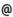## "Babes-Bolyai" University of Cluj-Napoca Faculty of Mathematics and Computer Science

 Functional analysis (2)
 Code Semes-ter Hours: C+S+L Credits Type Section MO005 6 2+1+0 5 compulsory Matematică MO005 6 2+1+0 4 optional Matematică-Informatică
 Teaching Staff in Charge
 Prof. COBZAS Stefan, Ph.D., scobzasmath.ubbcluj.ro
 Aims To present the basic results of the theory of topological vector spaces and of locally convex spaces and to deep some results on normed spaces, taught in the first semester.
 Content 1. Topological vector spaces (TVS). Definition and fundamental properties. The continuity of additive applications between TVS. Bases of neighborhoods of the origin. Linear functionals and hyperplanes in TVS. Seminormed spaces. Finite dimensional TVS - Tihonov's theorem. Topological properties of convex sets in TVS. Minkowski functional and seminorms. The continuity of Minkowski functional. Metrizability. Completeness, compactness and total boundedness in TVS. Note: Some questions concerning general topology as - the indroduction of a topology using neighborhood systems, nets and filters, topological products and Tihonov's theorem - will be treated at the seminars. 2. Locally convex spaces (LCS) The notion of LCS. The local convex toplogy generated by a family of seminorms. Locally convex bases, J. von Neumann's theorem. Bourbaki's theorem on the characterization of the continuity of linear applications between LCS. The existence of some continuous linear functionals on LCS. Weak topologies on normed spaces. The separation of convex sets by closed hyperplanes. Extremal points and Krein-Milman's theorem, Lyapunov convexity theorem. 3. Elements of distribution theory Inductive limits of LCS. The fundamental space of infinitely derivable functions. The notion of distribution. Regular distributions and singular distributions, distributions of finite order - examples.The topology of the space of distributions and the convergence of sequences of regular distributions. Applications of distribution theory to the solving of partial differential equations. 4. Fixpoint theorems Contractions and Banach fixpoint theorem. Fixpoint theorems for nonexpansive mappings. Theorems of Brouwer, Schauder-Tihonov and Markov-Kakutani. 5. Compact operators between normed spaces Arzela-Ascoli compactness criterium in C(T). Compact operators - fundamental properties. The conjugate of an operator. Schauder's theorem on the compactness of the conjugate of a compact operator. The spectrum of a compact operator, Riesz theory. 6. Operators on Hilbert spaces Symmetric operators, unitary operators, positive operators. The spectra of linear operators on Hilbert spaces.
 References 1. CRISTESCU R.: Notiuni de analiza functionala liniara. Bucuresti: Editura Academiei, 1998. 2. FABIAN M. et al.: Functional Analysis and Infinite-Dimensional Geometry. Berlin - New York: Springer Verlag, 2001. 3. KANTOROVICI L. V., AKILOV G. P.: Analiza functionala. Bucuresti: Editura Stiintifica si Enciclopedica, 1986. 4. KUTATELADZE S. S.: Fundamentals of Functional Analysis. Dordrecht: Kluwer Academic Publishers, 1995. 5. MEGGINSON R. E.: An Introduction to Banach Space Theory. Berlin - New York: Springer Verlag, 1998. 6. MUNTEAN I.: Analiza functionala. Capitole speciale. Cluj-Napoca: Universitatea Babes-Bolyai, 1990. 7. MUNTEAN I.: Analiza functionala. Cluj-Napoca: Universitatea "Babes-Bolyai", 1993 8. SCHAEFFER H. H., WOLF M. P.: Topological Vector Spaces. Berlin - New York: Springer Verlag, 1999.
 Assessment Exam.